Function Repository Resource:

# QuotientRule

See how to apply the quotient rule for derivatives to an expression

Contributed by: Wolfram|Alpha Math Team
 ResourceFunction["QuotientRule"][f,x] applies the quotient rule for computing the derivative of f with respect to x, returned as an inactive result.

## Details

ResourceFunction["QuotientRule"] accepts the following options:
 "PrintRule" False print a statement of the quotient rule "PrintNapkin" False print the side "napkin" word needed for computing components of the quotient rule “InactiveInnerDerivatives" False keep the derivatives of the components inactivated

## Examples

### Basic Examples (1)

See how to apply the quotient rule to compute the derivative of sin(x) /(8x) with respect to x:

 In:=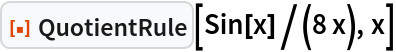Out=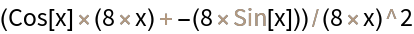### Scope (1)

In some cases, when there is no explicit quotient in the first argument of QuotientRule, the argument may be interpreted as a quotient:

 In:=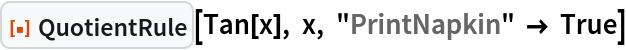Out=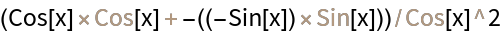### Options (3)

#### InactiveInnerDerivatives (1)

Setting "InactiveInnerDerivatives" to True inactivates the derivatives of the factors within the structure of the quotient rule:

 In:=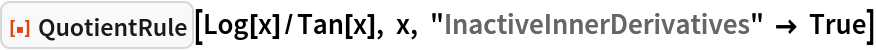Out=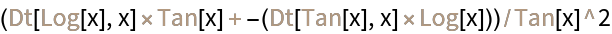#### PrintNapkin (1)

Setting "PrintNapkin" to True prints a grid with the side "napkin" work needed to apply the quotient rule (i.e., identifying and computing the derivatives of each factor):

 In:=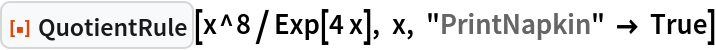Out=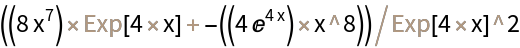#### PrintRule (1)

Setting "PrintRule" to True prints a general statement of the quotient rule for derivatives:

 In:=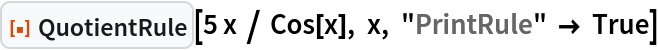Out=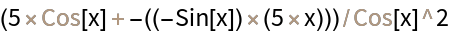## Publisher

Wolfram|Alpha Math Team

## Version History

• 2.0.0 – 23 March 2023
• 1.0.0 – 08 February 2023

## Author Notes

To view the full source code for QuotientRule, evaluate the following: